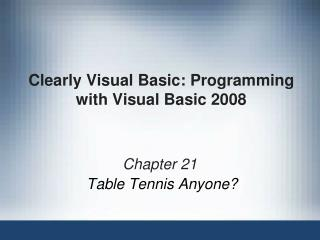DownloadDownload PresentationClearly Visual Basic: Programming with Visual Basic 2008

# Clearly Visual Basic: Programming with Visual Basic 2008

Download Presentation## Clearly Visual Basic: Programming with Visual Basic 2008

- - - - - - - - - - - - - - - - - - - - - - - - - - - E N D - - - - - - - - - - - - - - - - - - - - - - - - - - -
##### Presentation Transcript

1. Clearly Visual Basic: Programming with Visual Basic 2008 Chapter 21 Table Tennis Anyone?

2. Objectives • Create a two-dimensional array • Store data in a two-dimensional array • Search a two-dimensional array • Sum the values in a two-dimensional array Clearly Visual Basic: Programming with Visual Basic 2008

3. Let’s Table That Idea for Now • Two-dimensional array • Resembles a table in that the variables (elements) are in rows and columns • Each variable is identified by a unique combination of two subscripts • Variables located in the first row are assigned a row subscript of 0 • Variables in the second row are assigned a row subscript of 1 Clearly Visual Basic: Programming with Visual Basic 2008

4. Clearly Visual Basic: Programming with Visual Basic 2008

5. Let’s Table That Idea for Now (continued) • Figure 21-2 • Dim keyword • Used to create a procedure-level array • Private keyword • Used to create a module-level array • If array has two rows: • Statement that declares and initializes the array should have two initialValues sections • Use an outer loop and a nested loop: • To access each element in a two-dimensional array Clearly Visual Basic: Programming with Visual Basic 2008

6. Clearly Visual Basic: Programming with Visual Basic 2008

7. Clearly Visual Basic: Programming with Visual Basic 2008

8. Clearly Visual Basic: Programming with Visual Basic 2008

9. Clearly Visual Basic: Programming with Visual Basic 2008

10. Revisiting the Employee Application • Figure 21-4 • Shows planning information for the Employee application using a two-dimensional array • The values stored in an array • Come from a file on the computer’s disk • Are assigned to the array after it is declared Clearly Visual Basic: Programming with Visual Basic 2008

11. Clearly Visual Basic: Programming with Visual Basic 2008

12. The GetLowerBound and GetUpperBound Methods • Return an integer that indicates: • The lowest subscript and highest subscript, respectively, in the specified dimension in the array • Figure 21-6 • arrayName • Name of the array • dimension • Integer that specifies the dimension whose upper or lower bound you want to retrieve Clearly Visual Basic: Programming with Visual Basic 2008

13. Clearly Visual Basic: Programming with Visual Basic 2008

14. Calendar Orders Application • Displays the total number of calendars ordered by three stores in each of six months • Number ordered each month by each store • Stored in a three-row, six-column array Clearly Visual Basic: Programming with Visual Basic 2008

15. Clearly Visual Basic: Programming with Visual Basic 2008

16. Summary • Each element in a two-dimensional array • Identified by a unique combination of two subscripts: a row subscript and a column subscript • The first row subscript in a two-dimensional array is 0 • When declaring a two-dimensional array: • Provide either the highest row and column subscripts or the initial values • Number of rows in a two-dimensional array • One number more than its highest subscript Clearly Visual Basic: Programming with Visual Basic 2008

17. Summary (continued) • Refer to an element in a two-dimensional array • Using the array’s name followed by the element’s row and column subscripts • Use both an outer loop and a nested loop to: • Access each element in a two-dimensional array • Array’s GetLowerBound and GetUpperBound methods • Return the lowest subscript and highest subscript, respectively Clearly Visual Basic: Programming with Visual Basic 2008